While I always advocate keeping options trading simple, there are a few concepts that are necessary to comprehend in order to fully understand the variables that influence the price of an option.

These variables are commonly referred to as the “Greeks” and their purpose is to describe the main characteristics that influence risk and consequently the price of an option at any given time.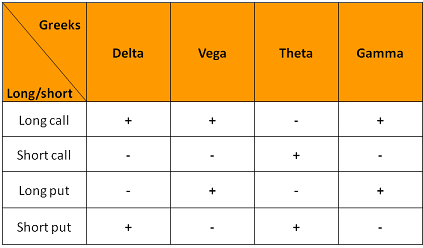By having a basic understanding of the different Greeks, an options trader can make better trading decisions, decrease risk and increase profitable trading opportunities.

The Greeks measure the sensitivity of the price of the option in relation to four 4 different factors: change in the underlying asset’s price, shifts in market volatility, rate of time decay and to a lesser degree interest rate fluctuations. The four Greeks that have the most impact on price include: Delta, Gamma, Theta and Vega. These Greeks are calculated using a theoretical options pricing model called the Black-Scholes model.

​Your ONLY Shot At 1,116x Returns…

There were 58 High Velocity Pullback entries over 12 months.

You’d have earned \$47,849 - or a one year return of 478%.

If those performance numbers hold up…

You could potentially multiply your account by 33.4x in two years.

In three years you’d potentially have a 193x multiple.

In four years - it might be 1,116x.

### Delta

Delta measures the amount an option’s price is expected to change for every \$1 change in the price of the underlying asset. For example, a Delta of 0.20 means that the option’s price will theoretically move \$0.20 for every \$1 move in the price of the underlying asset.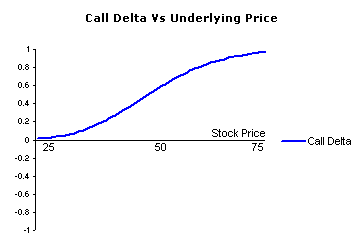Call options have delta ranging from zero to one, while put options have delta ranging from zero to negative one.

At the money call options usually have a delta of positive fifty while at the money put options delta is close to negative fifty.

The Delta of a call option that is trading in-the-money, will get closer to one as expiration approaches, while a Delta of a put option that’s trading in-the-money near expiration will have a Delta close to negative one.

The Delta of both a call option and a put option will begin moving closer to zero as expiration approaches.

The options Delta is often times used as a gauge to determine the probability that an option will expire in the money. To give you a simple example, if the Delta is .20, that option has roughly a .20% chance of ending up in the money at expiration.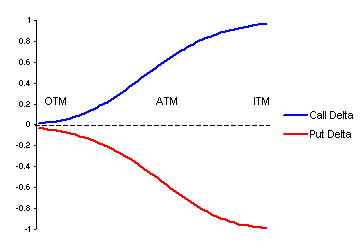Another common method of utilizing Delta is to determine how many shares need to be purchased or sold to match the exposure created by the option. If the Delta is .20 for example, for every dollar that the underlying asset moves either up or down, the option will shift about \$0.20; this information can become very useful in situations when options are being used as a hedge against risk to a stock portfolio.

For example, if you own \$100.00 shares of ABC stock and you want to protect against downside movement of \$10.00, by purchasing 2 contracts (each contract = 100 shares) of ABC put options that have a delta of 0.50.

If Delta was 0.25 instead 0.50, you would need 4 contracts instead of 2, to give enough protection in case ABC stock declined \$10.00.

### Gamma

Gamma is the sensitivity of the delta to changes in the price of the underlying asset. Gamma measures the change in the delta for every \$1 change in the underlying asset. In different words, Gamma let you know how much the option’s Delta should change as the price of the underlying asset moves either up or down.​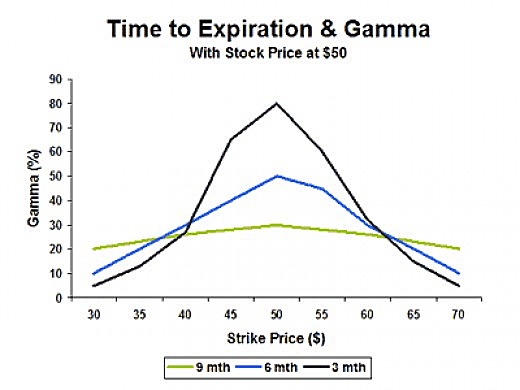In the example above you can see how Gamma reacts to three different time frames when the underlying asset is close to the strike price of the option. Notice that Gamma actually increases as time passes, as long as the option’s strike price is close to being at the money. When the asset moves much higher or lower.

There is a strong relationship between Delta and Gamma as the two are interdependent on each other. For example, if an option has a Delta of 0.50 and a Gamma of 0.10, if the underlying asset gained \$1.00 in value, the option would gain \$0.50 in value, but the Delta would now become 0.60. So the Gamma is used as a reference for the change in value of the Delta; similarly to how Delta is used to determine the change in the options price.

This Profit Window Will Be Glorious!

Volatility is plunging. Options are cheaper than they’ve been in months.

And, it’s all happening just as stocks have popped back out to the upside.

There has never been a better time to trade pullbacks with options.

It’s hunting season folks. On my webinar today - I’m giving you the rifles!

Attend the FREE training to learn how I do it.

### Theta

The theta value indicates how much value option’s price will diminish per day with all other factors being constant.  Simply put, Theta tells you how much the price of an option should decrease as the option nears expiration.

The nearer the expiration date, the higher the theta and the farther away the expiration date, the lower the theta.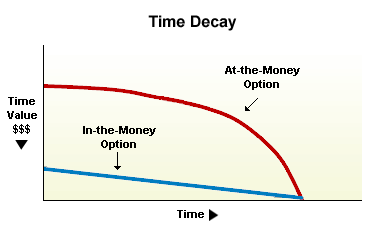If for example a call option with a current price of \$3 has a theta of -0.09, the option will lose \$0.09 per day and in two days the option would lose \$0.18 and would be now worth \$2.82. You should also note that options lose time value on weekends and holidays.

You should also keep in mind that option derived from stocks and ETFs that are highly volatile have higher Theta than options derived from stocks that are less volatile.

### Vega

Vega is the measurement of the option’s sensitivity to the change in the volatility of the underlying asset. It represents the amount that the options price shifts in reaction to a 1% change in the volatility of the underlying asset.

To give you a simple example of Vega, assume ABC stock is trading at \$50 in August and the September 55 call option is selling for \$3, the Vega of the option is 0.25 and the underlying volatility is 20%. If the volatility increases to 26%, the option will increase in price from \$3 to \$3.25. And if volatility would decrease from 25% to 24%, the option would decrease in price from \$3 to \$2.75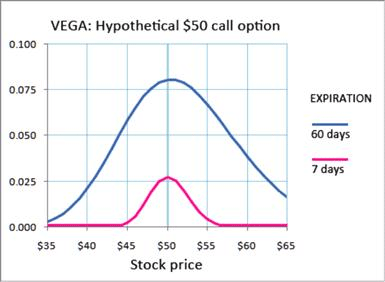As a general rule, Vega inflates when the option is at-the-money and gradually moves lower off as it goes either deeper in the money or further out of the money. Furthermore, as you can see from the chart above, volatility decreases substantially as the option gets closer to expiration since the probability of a substantial move is reduced greatly with each passing day.

Understanding Greeks can take much of the guesswork out of the various factors that influence the price of an options contract. By reviewing the Greeks before purchasing or selling an options contract, you will be in a position to choose the best option from a statistical perspective; this will give you an edge and help you gain a major advantage in your options trading.

All the best,

Roger Scott

Senior Publisher

WealthPress